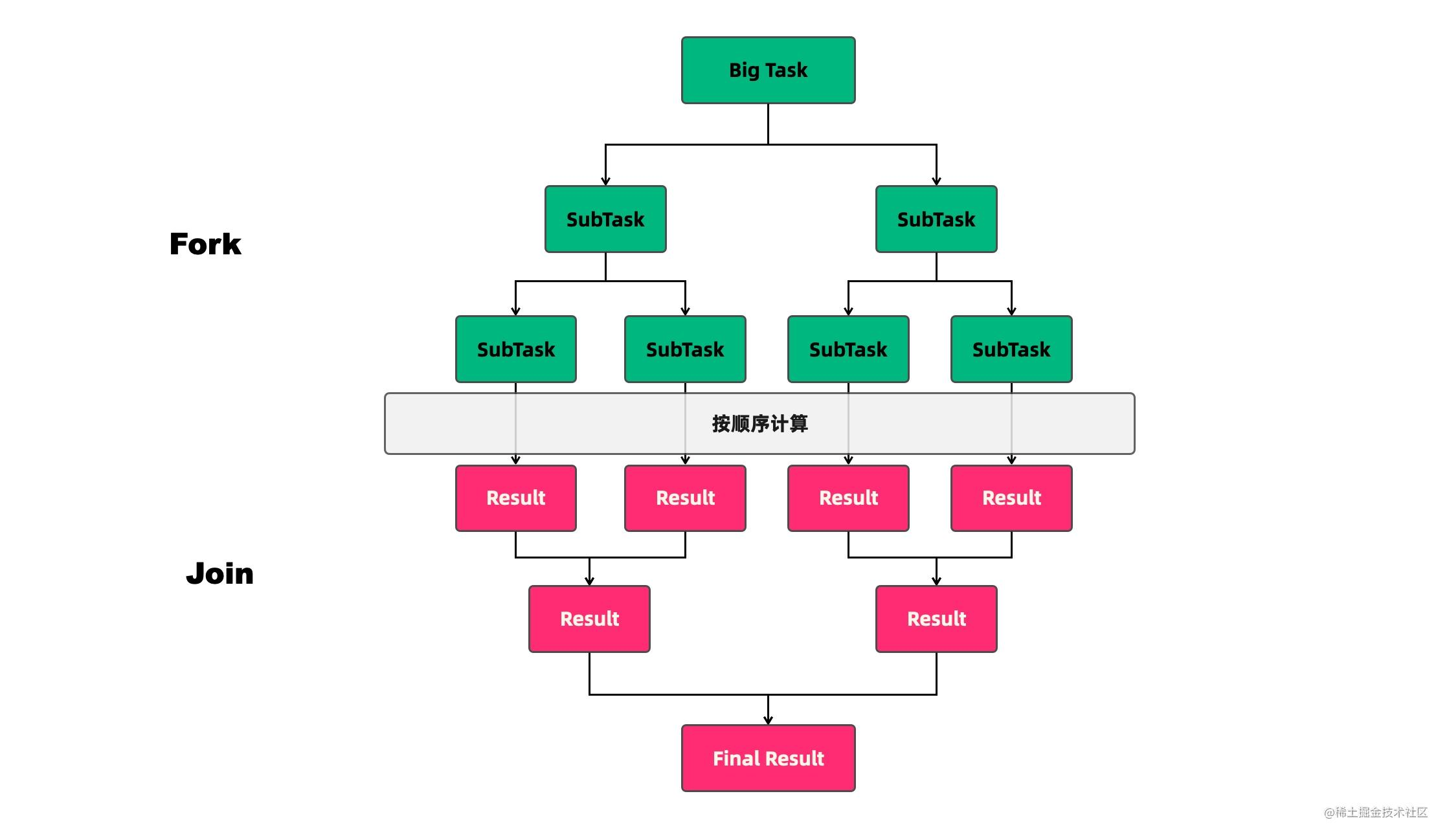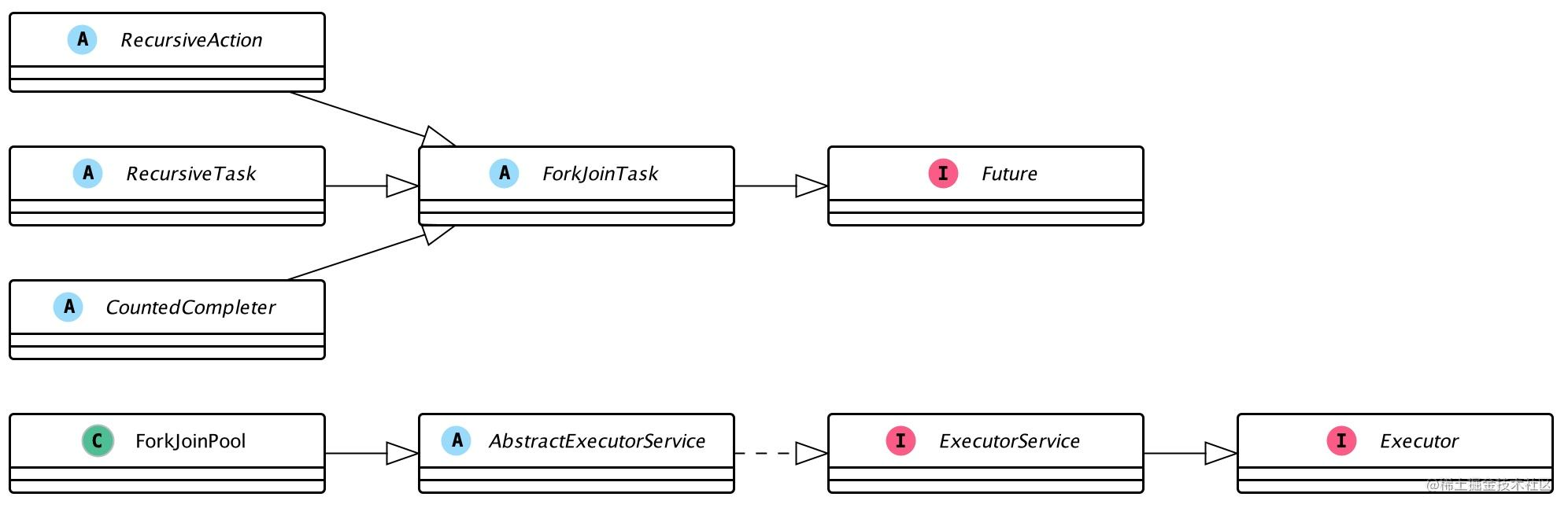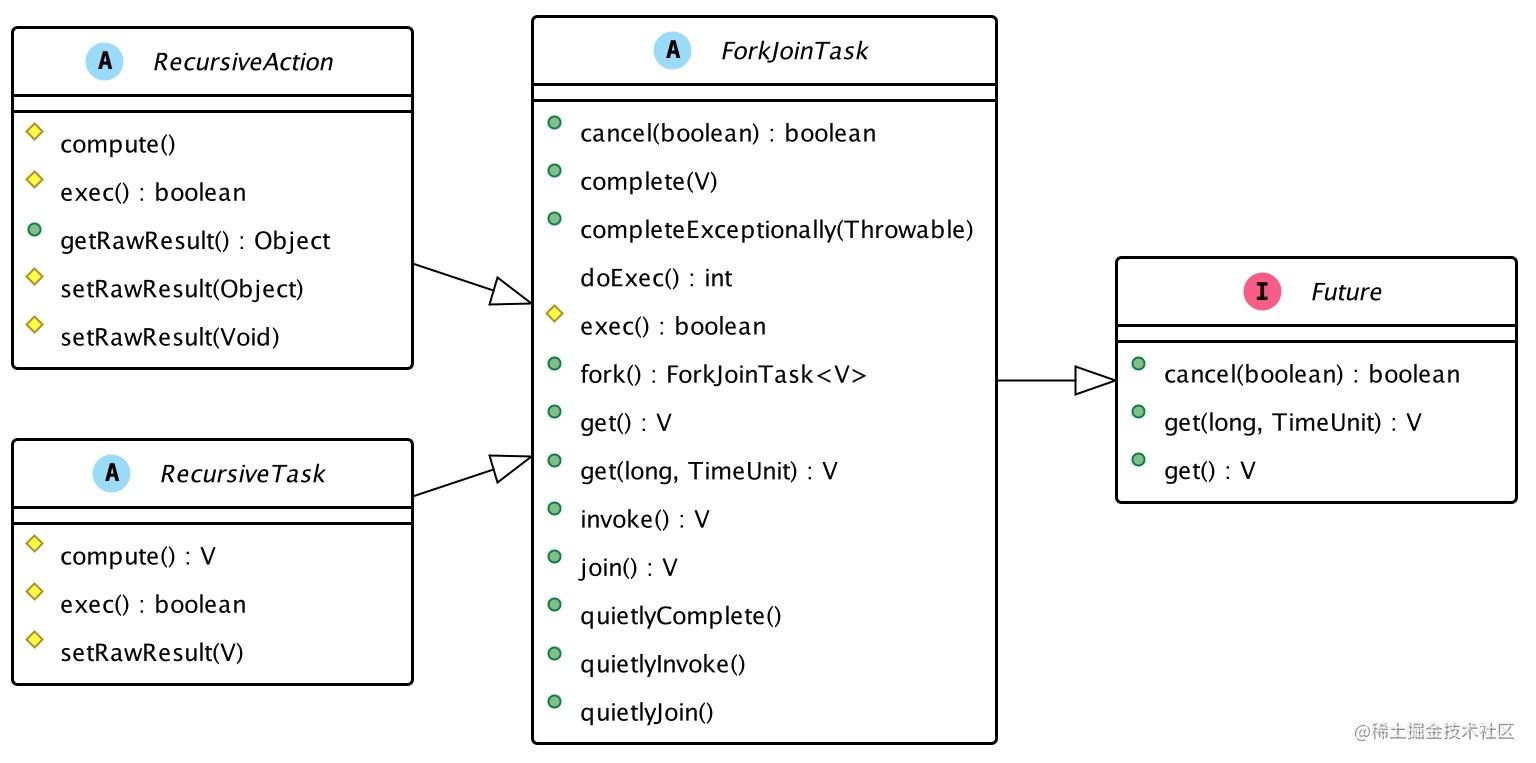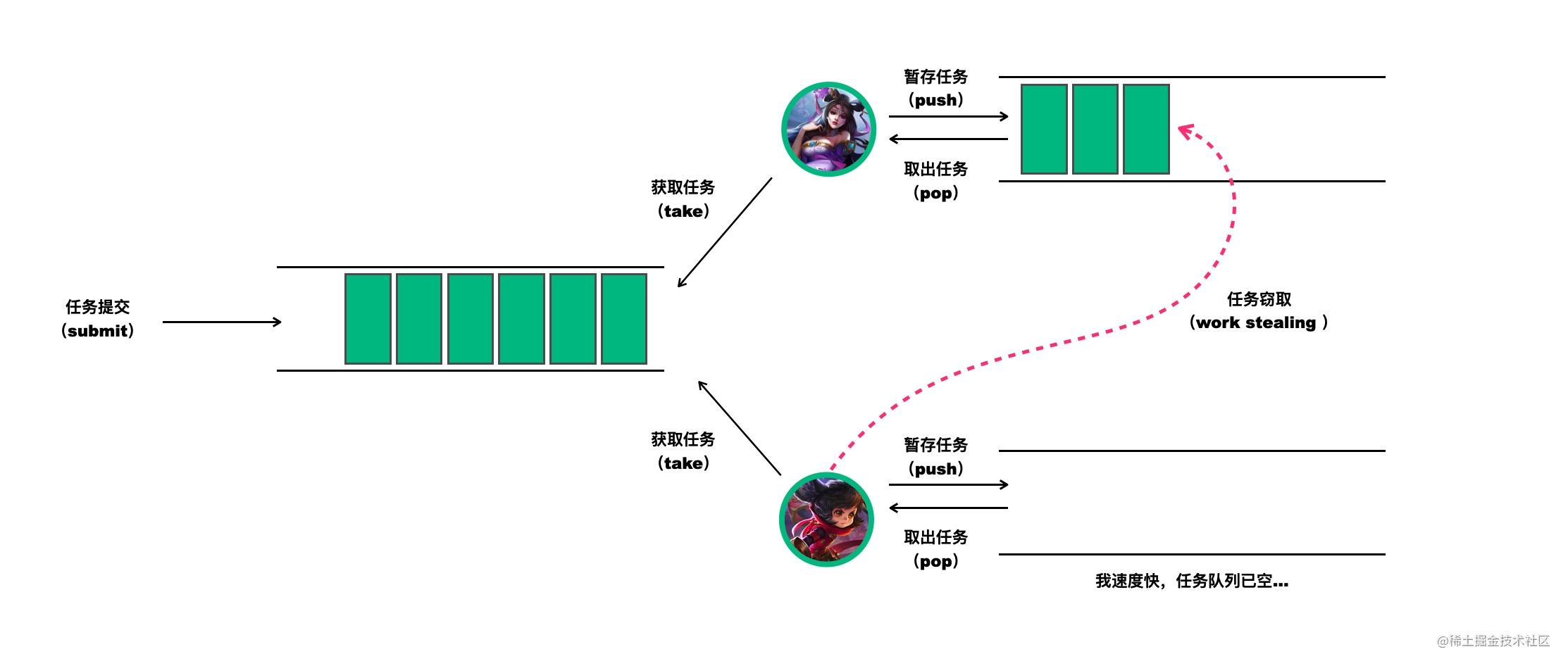# 王者并发课-钻石2：分而治之-如何从原理深入理解ForkJoinPool的快与慢

## 一、分治算法与Fork/Join模式

• （1）分解：将要解决的问题划分成若干规模较小的同类问题；
• （2）求解：当子问题划分得足够小时，用较简单的方法解决；
• （3）合并：按原问题的要求，将子问题的解逐层合并构成原问题的解。Fork/Join对任务的拆分和对结果合并过程也是如此，可以用下面伪代码来表示：

``````solve(problem):
if problem is small enough:
// 如果任务足够小，执行任务
solve problem directly (sequential algorithm)
else:
// 拆分任务
for part in subdivide(problem)
// 合并结果
join all subtasks spawned in previous loop
return combined results

## 二、Fork/Join应用场景与体验

TheKingRecursiveSumTask中定义了任务计算的起止范围（`sumBegin``sumEnd`）和拆分阈值（`threshold`），以及核心计算逻辑`compute()`.

``````public class TheKingRecursiveSumTask extends RecursiveTask<Long> {
private static final AtomicInteger taskCount = new AtomicInteger();
private final int sumBegin;
private final int sumEnd;
/**
* 任务拆分阈值，当任务尺寸大于该值时，进行拆分
*/
private final int threshold;

public TheKingRecursiveSumTask(int sumBegin, int sumEnd, int threshold) {
this.sumBegin = sumBegin;
this.sumEnd = sumEnd;
this.threshold = threshold;
}

@Override
protected Long compute() {
if ((sumEnd - sumBegin) > threshold) {
// 两个数之间的差值大于阈值，拆分任务
}
// 直接执行结果
long result = 0L;
for (int i = sumBegin; i < sumEnd; i++) {
result += i;
}
return result;
}

}
}

`````` public static void main(String[] args) {
int sumBegin = 0, sumEnd = 10000000;
computeByForkJoin(sumBegin, sumEnd);
}

private static void computeByForkJoin(int sumBegin, int sumEnd) {
ForkJoinPool forkJoinPool = new ForkJoinPool(16);
long forkJoinStartTime = System.nanoTime();
System.out.println("======");
System.out.println("ForkJoin计算结果：" + forkJoinResult);
System.out.println("ForkJoin计算耗时：" + (System.nanoTime() - forkJoinStartTime) / 1000000);
}

private static void computeBySingleThread(int sumBegin, int sumEnd) {
long computeResult = 0 L;
long startTime = System.nanoTime();
for (int i = sumBegin; i < sumEnd; i++) {
computeResult += i;
}
System.out.println("======");
System.out.println("单线程计算结果：" + computeResult);
System.out.println("单线程计算耗时：" + (System.nanoTime() - startTime) / 1000000);
}

``````======
ForkJoin任务拆分：131071
ForkJoin计算结果：49999995000000
ForkJoin计算耗时：207
======

Process finished with exit code 0

## 三、ForkJoinPool设计与源码分析### 1. 构造ForkJoinPool的几种不同方式

ForkJoinPool中有四个核心参数，用于控制线程池的并行数工作线程的创建异常处理模式指定等。各参数解释如下：

• `int parallelism`：指定并行级别（parallelism level）。ForkJoinPool将根据这个设定，决定工作线程的数量。如果未设置的话，将使用`Runtime.getRuntime().availableProcessors()`来设置并行级别；
• `ForkJoinWorkerThreadFactory factory`：ForkJoinPool在创建线程时，会通过factory来创建。注意，这里需要实现的是ForkJoinWorkerThreadFactory，而不是ThreadFactory. 如果你不指定factory，那么将由默认的DefaultForkJoinWorkerThreadFactory负责线程的创建工作；
• `UncaughtExceptionHandler handler`：指定异常处理器，当任务在运行中出错时，将由设定的处理器处理；
• `boolean asyncMode`：从名字上看，你可能会觉得它是异步模式设置，但其实是设置队列的工作模式：`asyncMode ? FIFO_QUEUE : LIFO_QUEUE`. 当asyncMode为true时，将使用先进先出队列，而为false时则使用后进先出的模式。

（1）方式一：默认无参构造

`````` public ForkJoinPool() {
this(Math.min(MAX_CAP, Runtime.getRuntime().availableProcessors()),
}

（2）方式二：通过并行数构造

`````` public ForkJoinPool(int parallelism) {
}

（2）方式三：自定义全部参数构造

``````public ForkJoinPool(int parallelism,
UncaughtExceptionHandler handler,
boolean asyncMode) {
this(checkParallelism(parallelism),
checkFactory(factory),
handler,
asyncMode ? FIFO_QUEUE : LIFO_QUEUE,
"ForkJoinPool-" + nextPoolId() + "-worker-");
checkPermission();
}

### 2. 按类型提交不同任务

（1）第一类核心方法：invoke

`````` public <T> T invoke(ForkJoinTask<T> task) {
throw new NullPointerException();
}

（2）第二类核心方法：execute

`````` public void execute(ForkJoinTask<?> task) {
throw new NullPointerException();
}

throw new NullPointerException();
else
externalPush(job);
}

（3）第三类核心方法：submit

``````   public < T > ForkJoinTask < T > submit(ForkJoinTask < T > task) {
throw new NullPointerException();
}

public < T > ForkJoinTask < T > submit(Callable < T > task) {
externalPush(job);
return job;
}

public < T > ForkJoinTask < T > submit(Runnable task, T result) {
externalPush(job);
return job;
}

throw new NullPointerException();
else
externalPush(job);
return job;
}#### （1）fork与join

`fork()`/`join()`是ForkJoinTask甚至是ForkJoinPool的核心方法，承载着主要的任务协调作用，一个用于任务提交，一个用于结果获取。

fork-提交任务

`fork()`方法用于向当前任务所运行的线程池中提交任务，比如上文示例代码中的`subTask1.fork()`. 注意，不同于其他线程池的写法，任务提交由任务自己通过调用`fork()`完成，对此不要感觉诧异，`fork()`内部会将任务与当前线程进行关联。

``````    public final ForkJoinTask<V> fork() {
else
ForkJoinPool.common.externalPush(this);
return this;
}

join-获取任务执行结果

``````public final V join() {
int s;
// 如果调用doJoin返回的非NORMAL状态，将报告异常
if ((s = doJoin() & DONE_MASK) != NORMAL)
reportException(s);
// 正常执行结束，返回原始结果
return getRawResult();
}

private int doJoin() {
int s;
ForkJoinPool.WorkQueue w;
//如果已完成，返回状态
return (s = status) < 0 ? s :
(w = (wt = (ForkJoinWorkerThread) t).workQueue).
tryUnpush(this) && (s = doExec()) < 0 ? s :
wt.pool.awaitJoin(w, this, 0 L):
externalAwaitDone();
}

final int doExec() {
int s;
boolean completed;
if ((s = status) >= 0) {
try {
completed = exec();
} catch (Throwable rex) {
return setExceptionalCompletion(rex);
}
// 执行完成后，将状态设置为NORMAL
if (completed)
s = setCompletion(NORMAL);
}
return s;
}RecursiveAction：无结果返回

RecursiveAction用于递归执行但不需要返回结果的任务，比如下面的排序就是它的典型应用场景。在使用RecursiveAction时，你需要继承并实现它的核心方法`compute()`.

``````static class SortTask extends RecursiveAction {
final long[] array;
final int lo, hi;
SortTask(long[] array, int lo, int hi) {
this.array = array;
this.lo = lo;
this.hi = hi;
}
this(array, 0, array.length);
}
// 核心计算方法
protected void compute() {
if (hi - lo < THRESHOLD)
// 直接执行
sortSequentially(lo, hi);
else {
// 拆分任务
int mid = (lo + hi) >>> 1;
merge(lo, mid, hi);
}
}
// implementation details follow:
static final int THRESHOLD = 1000;
void sortSequentially(int lo, int hi) {
Arrays.sort(array, lo, hi);
}
void merge(int lo, int mid, int hi) {
long[] buf = Arrays.copyOfRange(array, lo, mid);
for (int i = 0, j = lo, k = mid; i < buf.length; j++)
array[j] = (k == hi || buf[i] < array[k]) ?
buf[i++] : array[k++];
}
}

RecursiveTask用于递归执行需要返回结果的任务，比如前面示例代码中的求和或下面这段求斐波拉契数列求和都是它的典型应用场景。在使用RecursiveTask时，你也需要继承并实现它的核心方法`compute()`.

`````` class Fibonacci extends RecursiveTask<Integer> {
final int n;
Fibonacci(int n) { this.n = n; }
Integer compute() {
if (n <= 1)
return n;
Fibonacci f1 = new Fibonacci(n - 1);
f1.fork();
Fibonacci f2 = new Fibonacci(n - 2);
return f2.compute() + f1.join();
}
}

### 4. 工作队列与任务窃取

ForkJoinPool的大部分操作都发生在工作窃取队列（work-stealing queues ） 中，该队列由内部类WorkQueue实现。其实，这个队列也不是什么神奇之物，它是Deques的特殊形式，但仅支持三种操作方式：pushpoppoll（也称为窃取）。当然，在ForkJoinPool中，队列的读取有着严格的约束，`push``pop`仅能从其所属线程调用，而`poll`则可以从其他线程调用。换句话说，前两个方法是留给自己用的，而第三种方法则是为了方便别人来窃取任务用的。任务窃取的相关过程，可以用下面这幅图来表示，这幅图建议你收藏### 5. ForkJoinPool监控

（1）获取运行状态的线程总数

``````public int getRunningThreadCount() {
int rc = 0;
WorkQueue[] ws;
WorkQueue w;
if ((ws = workQueues) != null) {
for (int i = 1; i < ws.length; i += 2) {
if ((w = ws[i]) != null && w.isApparentlyUnblocked())
++rc;
}
}
return rc;
}

（2）获取活跃线程数量

``````
int r = (config & SMASK) + (int)(ctl >> AC_SHIFT);
return (r <= 0) ? 0 : r; // suppress momentarily negative values
}

（3）判断ForkJoinPool是否空闲

``````public boolean isQuiescent() {
return (config & SMASK) + (int)(ctl >> AC_SHIFT) <= 0;
}

（4）获取任务窃取数量

``````public long getStealCount() {
AtomicLong sc = stealCounter;
long count = (sc == null) ? 0 L : sc.get();
WorkQueue[] ws;
WorkQueue w;
if ((ws = workQueues) != null) {
for (int i = 1; i < ws.length; i += 2) {
if ((w = ws[i]) != null)
count += w.nsteals;
}
}
return count;
}

（5）获取队列中的任务数量

``````public long getQueuedTaskCount() {
long count = 0;
WorkQueue[] ws;
WorkQueue w;
if ((ws = workQueues) != null) {
for (int i = 1; i < ws.length; i += 2) {
if ((w = ws[i]) != null)
count += w.queueSize();
}
}
return count;
}

（6）获取已提交的任务数量

``````public int getQueuedSubmissionCount() {
int count = 0;
WorkQueue[] ws;
WorkQueue w;
if ((ws = workQueues) != null) {
for (int i = 0; i < ws.length; i += 2) {
if ((w = ws[i]) != null)
count += w.queueSize();
}
}
return count;
}

## 四、警惕ForkJoinPool#commonPool

ForkJoinPool中的commonPool设计初衷是为了降低线程池的重复创建，让一些任务共用同一个线程池，毕竟创建线程池和创建线程都是昂贵的。然而，凡事都有两面性，commonPool在某些场景下确实可以达到线程池复用的目的，但是，如果你决定与别人分享自己空间，那么当你想使用它的时候，它可能不再完全属于你。也就是说，当你想用commonPool时，它可能已经其他任务填满了。

## 五、ForkJoinPool性能评估

• 实验代码：本文第一部分的示例代码；
• 实验环境：Mac；
• JDK版本：8；
• 任务分隔阈值：100

Fork/Join 单线程 Fork/Join 单线程 Fork/Join 单线程
1 4 0 34 5 1157 313
2 3 0 34 6 848 344
3 5 0 16 9 1069 325
4 4 0 35 8 955 307
5 5 0 30 22 922 385

``````======
ForkJoin任务拆分：16383
ForkJoin计算结果：499999999500000000
ForkJoin计算耗时：143
======

## 小结

• 任务切分：将大的任务分割成更小粒度的小任务，让更多的线程参与执行；
• 任务窃取：通过任务窃取，充分地利用空闲线程，并减少竞争。

## 夫子的试炼

• 动手：使用ForkJoinPool实现List数组排序。